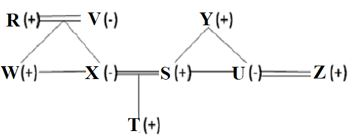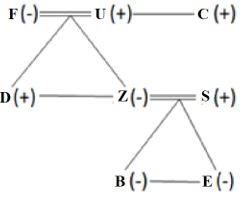Latest Banking jobs   »

# Reasoning Ability Quiz For SIDBI GRADE A 2023- 6th January

Q1. If all the letters in the word ORANGE are arranged in alphabetical order from left to right in such a way that one vowel is arranged followed by one consonant, then second vowel is arranged followed by consonants and so on. How many letters are there in between G and R after the arrangement?
(a) Three
(b) One
(c) None
(d) Two
(e) Five

Q2. If in the number 476592418, 5 is added to each of the digit which is less than three and 2 is subtracted from each of the digit which is greater than three then how many digits are repeating in the number thus formed?
(a) One
(b) Two
(c) none
(d) Four
(e) Three

Q3. Which of the following will be definitely true if the given expression W=L≥O=M>U≥C>H≥Q>P is definitely true?
(a) W <U
(b) L ≥ P
(c) P > M
(d) O ≥ H
(e) C < L

Q4. The position of how many alphabets will remain unchanged if each of the alphabets in the word ‘SIGNIFICANT’ is arranged in alphabetical order from left to right?
(a) Two
(b) Three
(c) One
(d) Four
(e) Five

Q5. Which of the following elements should come in a place ‘?’?
IR10   KP12   MN14   OL16   ?
(a) RS19
(b) RI19
(c) QR18
(d) QJ18
(e) None of these

Q6. How many pairs of letters are there in the word ‘TRADITIONAL’, each of which have as many letters between then in the word as they have between then in the English alphabet?
(a) More than Four
(b) One
(c) Three
(d) Two
(e) None

Q7. Adarsh ranked 25th from the top and 36th from the bottom in a class. How many students are there in the class?
(a) 61
(b) 59
(c) 52
(d) 58
(e) 60

Directions (8-11): Study the following data carefully and answer the questions accordingly.
There are nine members who belong to a family having three generations. S and U are siblings. U is the only daughter of Y and she is married. T is the nephew of W and Z has no child. Y is the father-in-law of X who is not a male. W is the son of R who is the husband of V. W is the brother of X. Z is the brother-in law of S.

Q8. How S is related to T?
(a) Grandfather
(b) Sister
(c) Uncle
(d) Father
(e) None of these

Q9. Who is the maternal grandfather of T?
(a) Y
(b) V
(c) R
(d) W
(e) Can’t be determined

Q10. How X is related to V?
(a) Son-in-law
(b) Daughter-in-law
(c) Daughter
(d) Sister
(e) None of these

Q11. How W is related to S?
(a) Son
(b) Brother
(c) Sister-in-law
(d) Brother-in-law
(e) None of these

Directions (12-15): Study the following data carefully and answer the questions accordingly.
A family has eight members B, C, D, E, F, S, Z, and U. There are 3 generations consisting of equal numbers of male and female members. There are two married couples. U’s brother has only one nephew and U is father of Z. D is unmarried and C has only one niece. D’s brother-in-law has two daughters. F’s son-in-law is married to Z. Z has only one sibling. U is father-in-law of S who have two daughters.

Q12. How many couples are there in the family?
(a) Four
(b) One
(c) Three
(d) Two
(e) Can’t be determined

Q13. Who among the following are the youngest family members?
(a) S, E
(b) Z, D
(c) B, E
(d) D, B
(e) None of these

Q14. Which of the following group represents the female members?
(a) Z, E, F, C
(b) F, D, S, E
(c) B, F, E, Z
(d) E, B, S, Z
(e) Can’t be determined

Q15. Four among the following are same in a certain way and forms a group, which among the following does not belong to the group?
(a) Son-in-law of F
(b) Brother-in-law of S
(c) Daughter of the sister of D
(d) Nephew of C
(e) Brother of U’s daughter

Solutions

S1. Ans. (a)
Original word- ORANGE
Obtained word- AGENOR

S2. Ans. (e)
Sol. Original Number- 476592418
Obtained Number- 254377266

S3. Ans. (e)

S4. Ans. (b)
Original word- SIGNIFICANT
Obtained word-ACFGIIINNST

S5. Ans. (d)

S6. Ans. (c)
Sol. LO, LN, NO

S7. Ans. (e)
Number of students in the class= 25+36-1=60

Solutions (8-11):
Sol.S8. Ans. (d)
S9. Ans. (c)
S10. Ans. (c)
S11. Ans. (d)

Solutions (12-15):
Sol.S12. Ans. (d)
S13. Ans. (c)
S14. Ans. (c)
S15. Ans. (c)## FAQs

### Q1. If all the letters in the word ORANGE are arranged in alphabetical order from left to right in such a way that one vowel is arranged followed by one consonant, then second vowel is arranged followed by consonants and so on. How many letters are there in between G and R after the arrangement? (a) Three (b) One (c) None (d) Two (e) Five

S1. Ans. (a)

#### Congratulations!